# ISEE Upper Level Quantitative : Variables

## Example Questions

1 2 3 4 5 6 7 8 9 11 Next →

### Example Question #2 : How To Divide Exponential Variables

Simplify: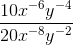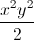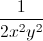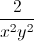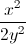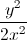Explanation:

Break the fraction up and apply the quotient of powers rule: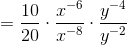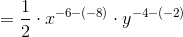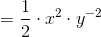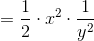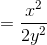### Example Question #42 : Variables And Exponents

Simplify: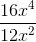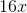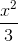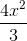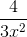Explanation:

To simplify this expression, look at the like terms separately. First, simplify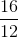. This becomes. Then, deal with the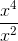. Since the bases are the same and you're dividing, you can subtract exponents. This gives you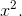Since the exponent is positive, you put in the numerator. This gives you a final answer of.

### Example Question #43 : Variables And Exponentsis a negative number.

Which is the greater quantity?

(a) The reciprocal of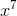(b) The reciprocal of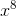(a) and (b) are equal

(a) is the greater quantity

(b) is the greater quantity

It is impossible to determine which is greater from the information given

(b) is the greater quantity

Explanation:

A negative number raised to an odd power is negative; a negative number raised to an even power is positive. It follows thatis negative andis positive. Also, the reciprocal of a nonzero number assumes the same sign as the number itself, so the reciprocal ofis positive and that ofis negative. It follows that the reciprocal ofis the greater of the two.

### Example Question #51 : Variables And Exponents

Simplify:Explanation:

Break the fraction up and apply the quotient of powers rule:1 2 3 4 5 6 7 8 9 11 Next →

### All ISEE Upper Level Quantitative Resources